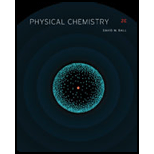# Show that s → s transitions are not allowed in the hydrogen atom. To do this, show that the integral ∫ Ψ a ( s ) * O ⌢ Ψ b ( s ) d τ is exactly zero where O ⌢ is the operator representing the light. Assume that light has the symmetry species D u ( 1 ) in this completely spherical system.### Physical Chemistry

2nd Edition
Ball + 3 others
ISBN: 9781133958437

#### Solutions

Chapter
Section### Physical Chemistry

2nd Edition
Ball + 3 others
ISBN: 9781133958437
Chapter 13, Problem 13.66E
Textbook Problem
1 views

## Show that s → s transitions are not allowed in the hydrogen atom. To do this, show that the integral ∫ Ψ a ( s ) * O ⌢ Ψ b ( s )   d τ is exactly zero where O ⌢ is the operator representing the light. Assume that light has the symmetry species D u ( 1 ) in this completely spherical system.

Interpretation Introduction

Interpretation:

The given statement that ss transitions are not allowed in the hydrogen atom is to be validated. The value of integral Ψa(s)*OΨb(s)dτ is exactly equal to zero is to be stated.

Concept introduction:

The group of symmetry operations of which atleast one point is kept fixed is called point group. The symmetry operations can be identity, rotation, reflection, inversion and improper rotation.

### Explanation of Solution

The symmetry of hydrogen is described by the Rh(3) point group. The irreducible representation of 1s orbital is represented by Dg(0). The irreducible representation of 2s orbital is represented by Dg(0). The light is represented by Du(1) symmetry species. It causes an electron to excite from 1s state to other state.

The table is taken from the Rh(3) character table as shown below.

ECϕiSϕσDg(0)11111Dg(0)11111Du(1)

### Still sussing out bartleby?

Check out a sample textbook solution.

See a sample solution

#### The Solution to Your Study Problems

Bartleby provides explanations to thousands of textbook problems written by our experts, many with advanced degrees!

Get Started

Find more solutions based on key concepts
Describe diffusion in terms of entropy.

BIOLOGY:CONCEPTS+APPL.(LOOSELEAF)

To be labeled "low fat. a food must contain 3 grams of fat or less per serving. T F

Nutrition: Concepts and Controversies - Standalone book (MindTap Course List)

List the basic types of membranes in the body.

Human Biology (MindTap Course List)

Unlike mass, weight is not a(n) ___ property. (1.4)

An Introduction to Physical Science

Identify a genetic condition that is not found by maternal or newborn screening.

Nutrition Through the Life Cycle (MindTap Course List)

Why did Newton conclude that gravity has to be mutual and universal?

Foundations of Astronomy (MindTap Course List)

Consider a solenoid of length and radius a containing N closely spaced turns and carrying a steady current I. ...

Physics for Scientists and Engineers, Technology Update (No access codes included)

What causes global warming?

Oceanography: An Invitation To Marine Science, Loose-leaf Versin